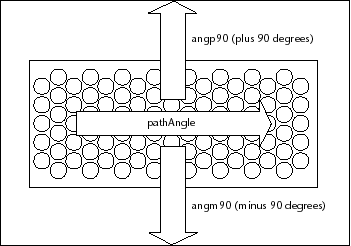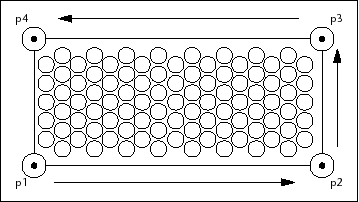# Using Angles and Setting Up Points

Using Angles and Setting Up Points

There are still a couple of issues remaining. First, you need to figure out how to draw the path at any angle the user specifies. From the gp:getPointInput function, you can easily establish the primary angle of the path. To draw it, you need a couple of additional vectors perpendicular to the primary angle.This is where the Degrees->Radians function is useful. The following code fragment demonstrates how you can set up your two perpendicular vectors using the PathAngle variable as an argument passed to the Degrees->Radians function:

`(setq angp90 (+ PathAngle (Degrees->Radians 90))`
`      angm90 (- PathAngle (Degrees->Radians 90)))`

With the data you now have in hand, you can establish the four corner points of the path using polar function:`(setq p1 (polar StartPt angm90 HalfWidth)`
`      p2 (polar p1 PathAngle PathLength)`
`      p3 (polar p2 angp90 Width)`
`      p4 (polar p3 (+ PathAngle (Degrees->Radians 180))`

The polar function returns a 3D point at a specified angle and distance from a point. For instance, polar locates p2 by projecting p1 a distance of PathLength along a vector oriented at an angle of PathAngle, counter-clockwise from the x-axis.